Fundamentals of Digital Image Processing

A Practical Approach with Examples in Matlab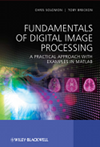Supporting materials

For Students: electronic copies of all of the chapter figures, Matlab program code examples and copies of digital images used in the book examples are made available from this page. In addition links to external open source Matlab code material that may further aid your understanding are made where appropriate.

For Instructors: additional high resolution copies of all of the book figures/tables/equations are available from the figures gallery and eqn/tables gallery for use on course slides. Additional examples can readily be generated from the supplied Matlab program code examples (below) and electronic copies of the chapter exercises are also provided in addition to suggested extended project exercises.

A general Introduction to Matlab Primer is also available for students and instructors alike.

Materials Index: [ Images] [ 1]  [ 3]  [ 5]  [ 7]  [ 9]  [ 11]
[Matlab] [ Projects][Instructors] [ Errata]

Sample Image Gallery

Several of these images originate from the Matlab Image Processing Toolkit (IPT) and have copyright assigned as follows - IPT image credits notice. They are included here for reader convenience in PNG format under 'fair use' for research/teaching/scholarship purposes.

Note that the original image files and references in book text/examples may be TIF format for some examples -i.e. change filename.tif references to filename.png to use these images with the book examples where needed. A full set of original TIFF images are made available as a zip archive.

Chapter 1 - Representation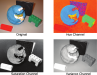Chapter 2 - Formation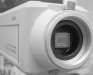Chapter 3 - PixelsChapter 4 - Enhancement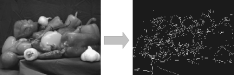• chapter contents
• Chapter Figures (EPS/PNG format) - [ZIP]
• Chapter Matlab Program Code Examples (.m files) - [GitHub]
• Chapter Example Images - [ZIP]

Chapter 5 - Linear systems and frequency-domain processing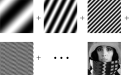Chapter 6 - Image restoration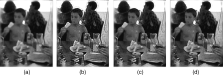Chapter 7 - Geometry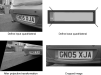Chapter 8 - Morphological processing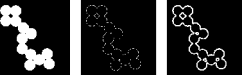Chapter 9 - Features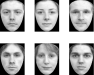Chapter 10 - Image SegmentationChapter 11 - Classification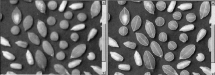Matlab Resources

• Matlab is available directly from Mathwork Inc. To use the examples/exercises in this text you require a both Matlab and the Image Processing Toolbox (IPT).

Matlab (student version for study use) from Amazon: UK | US | CA | FR | DE | IT | JP
Student version also available from a range of other national/international retailers.
• Getting Started with Matlab Primers (for students or instructors alike):

Extended Project Resources

Two extended "challenge" type exercises are provided for the reader to try out their image processing skills (and programming) on a real world image processing problems drawn from the context of automated industrial inspection in manufacturing. Please note an example solutions to these problems are not provided.

Instructors: These may be suitable as extended assignments or exercises for your course. Please see corresponding PDF for details of additional exemplar/support materials.

•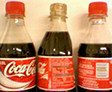Project 1: Industrial Inspection - soft drink bottles
An automated industrial inspection task for the identification of faults in soft drink bottles leaving a production facility - [PDF]

Matlab: [dir_cycle.m]
Data: All images [All]
Subsets (fault) [ 1] [ 2] [ 3] [ 4] [ 5] [ 6] [ 7] [ Combined] [ Missing] [ Side] [ No Fault]

•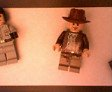Project 2: Industrial Inspection - indy toy characters
An automated industrial inspection task for the identification of faults in toy characters leaving a production facility - [PDF]

Matlab: [dir_cycle.m]
Data: All images [All]
Subsets (fault) [ 1] [ 2] [ 3] [ 4] [ 5] [ 6] [ 7] [ Combined] [ Other] [ No Fault]

Instructor Resources

• Instructors: Electronic Copies of Chapter Exercises (as PDF):
[ 1] [ 2] [ 3] [ 4] [ 5] [ 6] [ 7] [ 8] [ 9] [ 10] [ 11]

Suggested student work-plan:- Matlab Primer (here), chapters 1-4 (Matlab Programming), chapters 5-11 (mixture of paper-based Exam prep. type questions and Matlab Programming), one or both provided student projects as potential course assignments or extended exercises ( here).
• Instructors: (course slide materials)

Errata

• Chapter 1: p4 (Bit resolution) "...quantization level, e.g. binary is 2-bit..." should of course read "...quantization level, e.g. binary is 1-bit..." as to distinguish between black and white requires only 1 bit which gives us the 2 colour levels (0 / 1).
• Chapter 1: p. 15 (Example 1.2) - added the use of subplot() to show both images if ran as a script
This is now corrected in the on-line version of the Matlab code [GitHub].
• Chapter 1: p. 16/17 (Example 1.5) - incorrect use of imview instead of imshow and incorrect notation for setting pixel value
This is now corrected in the on-line version of the Matlab code [GitHub].
• Chapter 1: Referenced colour plate Figure 1.10 should use the label "Value channel" in place of "Variance channel" to be consistent with the text and with colour plate Figure 1.9 earlier.
• Chapter 2: p. 32 (Figure 2.9) - within the in-line mathematics caption for Figure 2.9c the second line should be hd(x') instead of H(x,)
• Chapter 2: p. 35 (Figure 2.12) - the equation within the figure should be replaced with Equation 2.20 where the summation variables j and i are defined for the ranges {0..2}. As a result, the output of the convolution at a given pixel location (as shown) becomes gkl over input image f.
• Chapter 3: p. 58 (Equation 3.5) - for Ioutput to be maximally 255, c should in fact be:
c = 255/(ln(1+(esigma-1)max(Iinput)))
i.e. the (esigma-1) should be added to re-scale max(Iinput) and the log()) should be changed to natural log ln()) to be consistent with the formulation shown in Equation 3.4.
• Chapter 3: p. 67 (Equation 3.10) - equation should be:
Ioutput(i,j) = (Iinput(i,j) - d)((a-b)/(c-d)) + b
based on the definitions of (a,b,c,d) given the text as (i.e. a = intended output maximum (255), b = intended output minimum (0) and c / d are the maximum / minimum pixel values currently present in the input image, Iinput. Firstly, we remove the offset in the input by subtracting the minimum value, not the maximum. Secondly, we add the new minimum to the scaled value, not the new maximum.
• Chapter 3: p. 68 (Example 3.18) - the subplot indices are incorrect for the correct reproduction of Figure 3.16.
This is now corrected in the on-line version of the Matlab code [GitHub].
• Chapter 3: p. 71 (Equation 3.15) - the summation variable j is currently unused in the equation presented. Inside the summation operator should be px(xj) instead of px(xk).
• Chapter 3: p. 72 (Equation 3.20) - C(Input(c,r) is currently missing a closing parenthesis and should be C(Input(c,r)) to be consistent with C(i) = C(Input(c,r)) in the expansion of Equation 3.19 above into our familiar image based notation.
• Chapter 3: p. 74 (Equation 3.23) - the variable names do not coincide with the text above. Equation 3.23 should be f(x) = dp/dX|_X=x (N.B. 2 changes - p(x) to f(x) and uppercase P to lowercase p)
• Chapter 5: p. 131 (Equations 5.16 and 5.17) - both equations use the "**" notation for the convolution operator which is inconsistent with the earlier use of a single operator "*" for the same operation.
• Chapter 5: p. 131 (Equations 5.17 and 5.18) - h(x,y) is missing its closing parenthesis in both equations such that ..h(x,y} should be ..h(x,y)} in both instances.
• Chapter 6: p. 148 (Equations 6.11) - this equation should be written in using lowercase ~{f} and f to be consistent with the text above.
• Chapter 6: p. 148 (Equations 6.12) - the integral and arguments should be kx and ky, for consistency with the rest of the notation in this section.
• Chapter 6: p. 148 (Equations 6.13) - the integration constants should be dkx and dky, for consistency with the rest of the notation in this section.
• Chapter 6: p. 152 (Equations 6.15 and 6.16) - the use of bold font on L within the equation is erroneous (although is consistent with the text above).
• Chapter 7: p. 174 (Table 7.1) - in the last row the first s symbol (coresponding to the coefficient a_(11) should be 1.
• Chapter 7: p. 176 (Equation 7.14) - the greek symbol beta should in fact be gamma for consistency with Equation 7.12.
• Chapter 7: p. 177 (Equation 7.15) - the matrix on the right is meant to be labelled as variable Y (not XY).
• Chapter 7: p. 178 (Equation 7.23) - the symbol for the diagonal matrix S clashes with the use of the same fr scaling matrix S above but both are in fact distinct.
• Chapter 9: p. 245 (Example 9.3) -
1 ) The commented out line "I=double(bw)./(sum(sum(bw)));" appears erroneously and should not be present.
2) The calculation of normalised central momment eta_03 erroneously uses central moment M_02 when it should be M_03.
This is now corrected in the on-line version of the Matlab code [GitHub].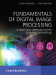The authors would like to fully thank all contributors to the errata list who take the time to carefully report issues they encounter with the text.

In addition, a notable special thanks to the work of Jon Sporring and his students at University of Copenhagen for their thorough work on reviewing the text through its use in their classes.

Without this valuable feedback, this text and its associated resources would be nowhere near as strong as they are.